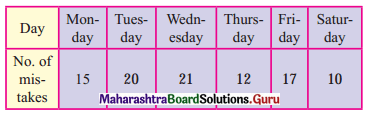# Maharashtra Board 11th Maths Solutions Chapter 8 Measures of Dispersion Ex 8.1

Balbharti Maharashtra State Board Class 11 Maths Solutions Pdf Chapter 8 Measures of Dispersion Ex 8.1 Questions and Answers.

## Maharashtra State Board 11th Maths Solutions Chapter 8 Measures of Dispersion Ex 8.1

Question 1.
Find a range of the following data:
19, 27, 15, 21, 33, 45, 7, 12, 20, 26
Solution:
Here, largest value (L) = 45, smallest value (S) = 7
∴ Range = L – S = 45 – 7 = 38

Question 2.
Find range of the following data:
575, 609, 335, 280, 729, 544, 852, 427, 967, 250
Solution:
Here, largest value (L) = 967, smallest value (S) = 250
∴ Range = L – S = 967 – 250 = 717Question 3.
The following data gives a number of typing mistakes done by Radha during a week. Find the range of the data.Solution:
Here, largest value (L) = 21, smallest value (S) = 10
∴ Range = L – S = 21 – 10 = 11

Question 4.
The following results were obtained by rolling a die 25 times. Find the range of the data.Solution:
Here, largest value (L) = 6, smallest value (S) = 1
∴ Range = L – S = 6 – 1 = 5Question 5.
Find range for the following data:Solution:
Here, upper limit of the highest class (L) = 72
lower limit of the lowest class (S) = 62
∴ Range = L – S = 72 – 62 = 10